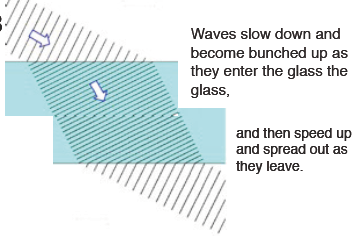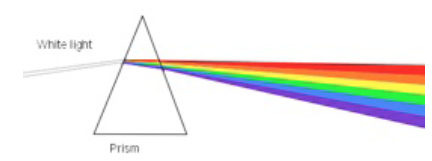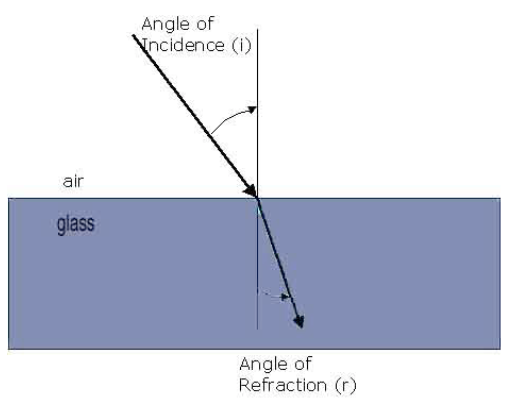### Refractionrefraction of waves

Refraction occurs when light passes from one medium to another, for example from air to glass or water to air or the reverse. The reason is that the light slows down when it enters a denser medium (and speeds up when it leaves). In the diagram we can see that this makes the direction of the waves alter.

Waves slow down and become bunched up as they enter the glass the glass and then speed up and spread out as they leave.

When we pass light through a shape where the sides are not parallel, as in this prism, we get a spectrum. Because the speed the different waves, which we see as colour, travel at different speed in dense material. (They all travel at the same speed in a vacuum and almost the same in air). As a consequence the different waves/ colours in white light refract differently. Blue slows the most and is refracted the most, red refracts the least and so the white light is split up.spectrum forms

### Snell’s Law

The connection between the angles as the light bends is called “Snell’s Law”.

Snell's Law states that ratio of the sine of the angle of incidence to the sine of the angle of refraction is a constant for any pair of materials. It is called the refractive index, μ.

The refractive index is also equal to the ratio of speeds, so in the example of this glass block:

sin i/sin r  = μ = speed of light in air/speed of light in glass

where μ is the refractive index for air to glass. Typical values for μ for air to glass are between 1.45 and 1.8. For air to water μ is 1.33 meaning that light travels 1.33 times faster in a vacuum as it does in water.refraction

Other pages on the physics of light which you may find useful are:

Total internal reflection and optical fibres - notes and video lesson

Drawing ray diagrams for lenses - notes and video lessons